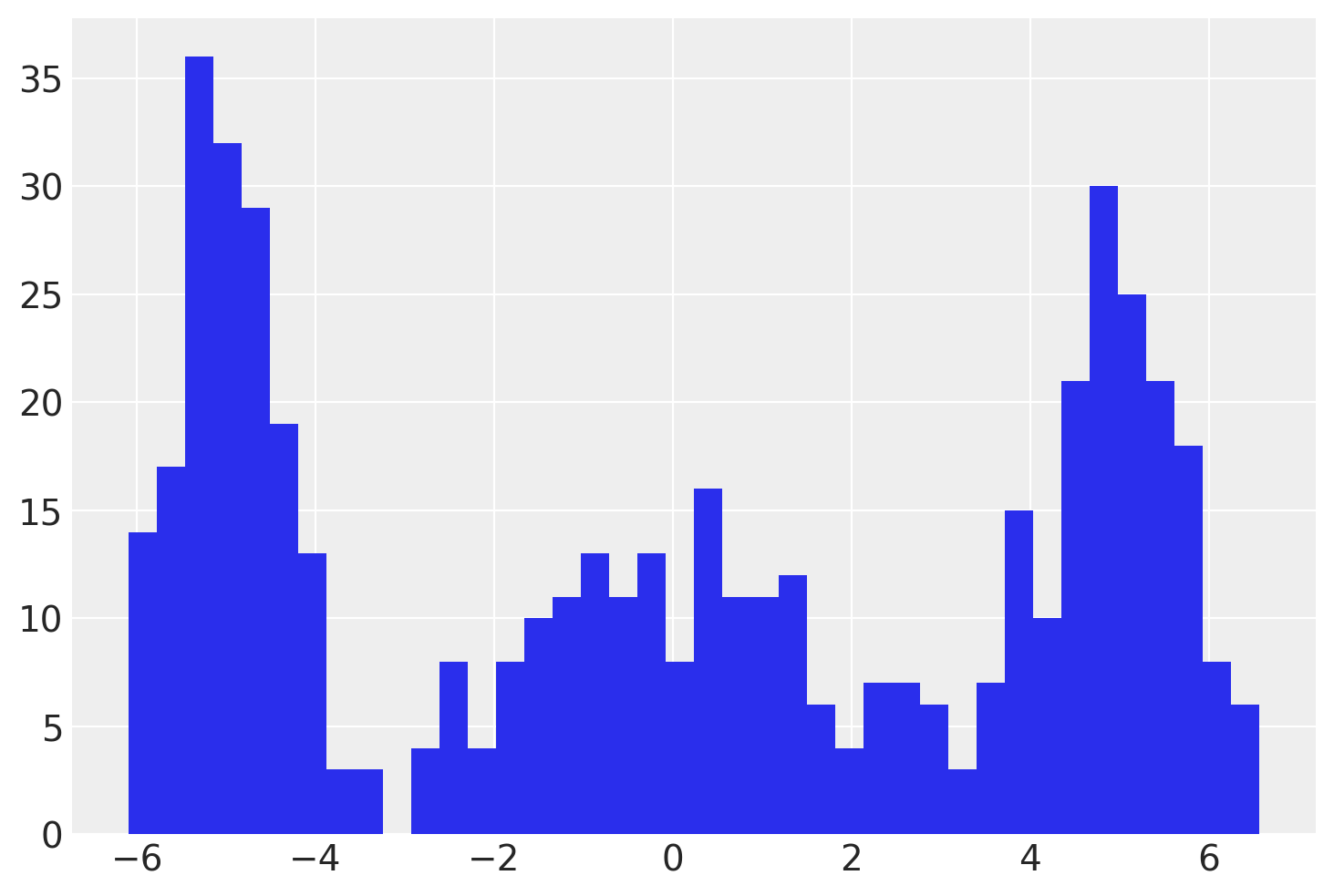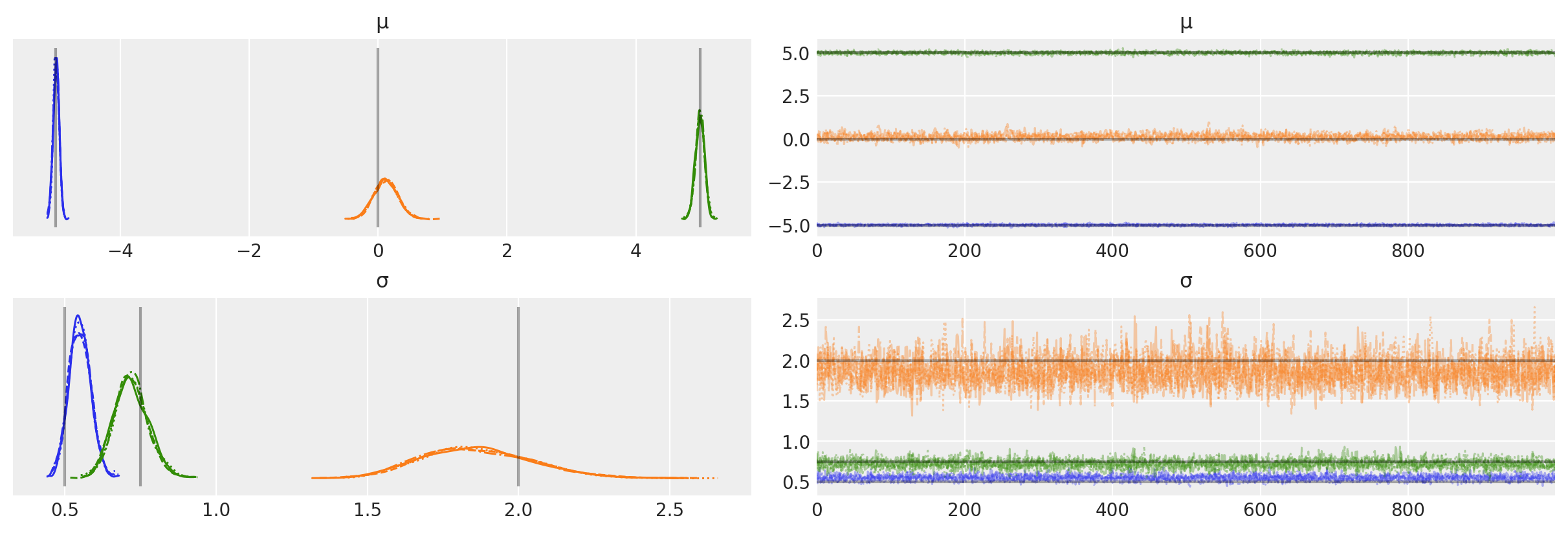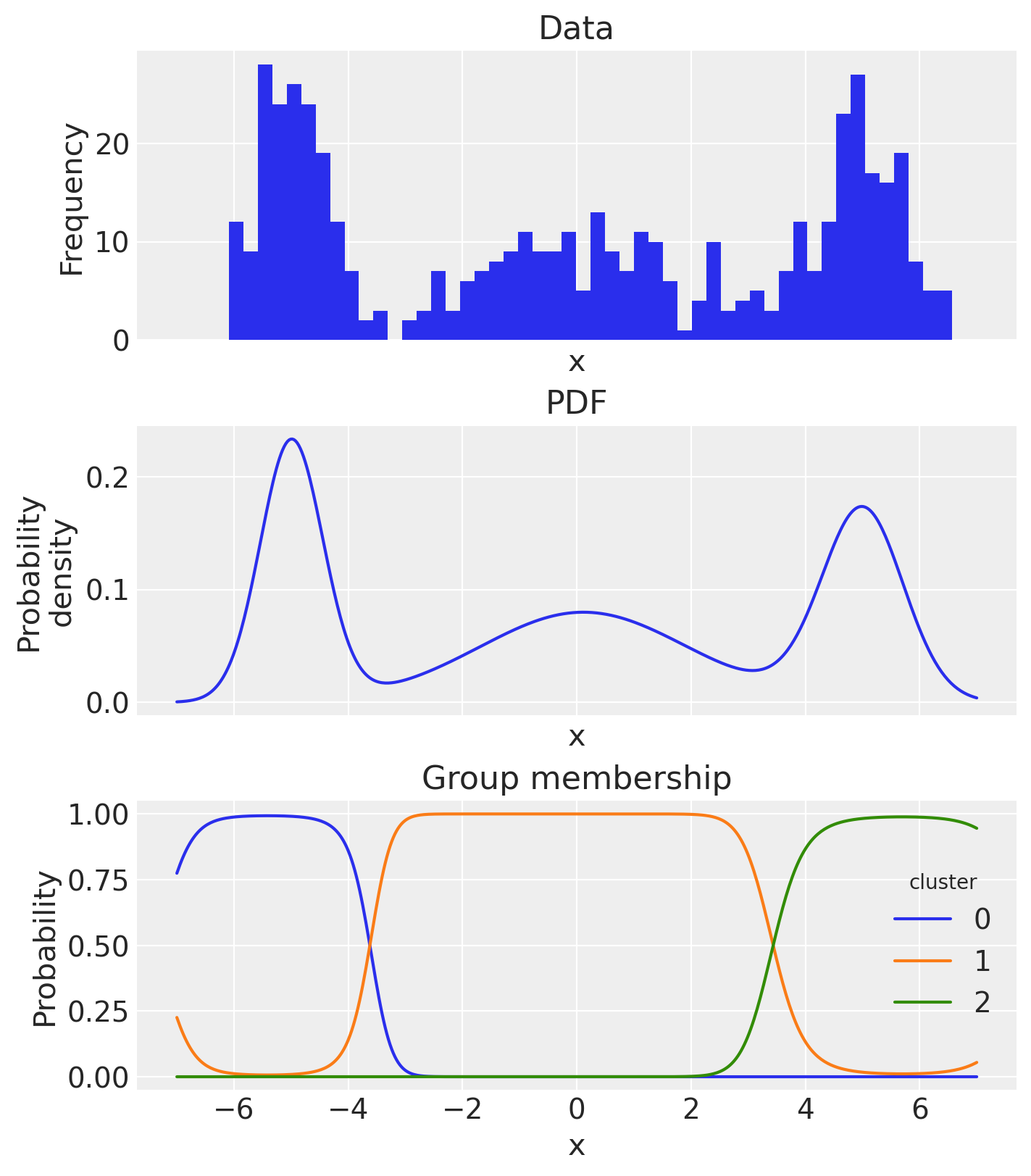# Gaussian Mixture Model#

A mixture model allows us to make inferences about the component contributors to a distribution of data. More specifically, a Gaussian Mixture Model allows us to make inferences about the means and standard deviations of a specified number of underlying component Gaussian distributions.

This could be useful in a number of ways. For example, we may be interested in simply describing a complex distribution parametrically (i.e. a mixture distribution). Alternatively, we may be interested in classification where we seek to probabilistically classify which of a number of classes a particular observation is from.

import arviz as az
import matplotlib.pyplot as plt
import numpy as np
import pandas as pd
import pymc as pm

from scipy.stats import norm
from xarray_einstats.stats import XrContinuousRV

%config InlineBackend.figure_format = 'retina'
RANDOM_SEED = 8927
rng = np.random.default_rng(RANDOM_SEED)
az.style.use("arviz-darkgrid")


First we generate some simulated observations.

Hide code cell source
k = 3
ndata = 500
centers = np.array([-5, 0, 5])
sds = np.array([0.5, 2.0, 0.75])
idx = rng.integers(0, k, ndata)
x = rng.normal(loc=centers[idx], scale=sds[idx], size=ndata)
plt.hist(x, 40);In the PyMC model, we will estimate one $$\mu$$ and one $$\sigma$$ for each of the 3 clusters. Writing a Gaussian Mixture Model is very easy with the pm.NormalMixture distribution.

with pm.Model(coords={"cluster": range(k)}) as model:
μ = pm.Normal(
"μ",
mu=0,
sigma=5,
transform=pm.distributions.transforms.univariate_ordered,
initval=[-4, 0, 4],
dims="cluster",
)
σ = pm.HalfNormal("σ", sigma=1, dims="cluster")
weights = pm.Dirichlet("w", np.ones(k), dims="cluster")
pm.NormalMixture("x", w=weights, mu=μ, sigma=σ, observed=x)

pm.model_to_graphviz(model)with model:
idata = pm.sample()

Auto-assigning NUTS sampler...
Multiprocess sampling (4 chains in 4 jobs)
NUTS: [μ, σ, w]

100.00% [8000/8000 00:03<00:00 Sampling 4 chains, 0 divergences]
Sampling 4 chains for 1_000 tune and 1_000 draw iterations (4_000 + 4_000 draws total) took 4 seconds.


We can also plot the trace to check the nature of the MCMC chains, and compare to the ground truth values.

az.plot_trace(idata, var_names=["μ", "σ"], lines=[("μ", {}, [centers]), ("σ", {}, [sds])]);And if we wanted, we could calculate the probability density function and examine the estimated group membership probabilities, based on the posterior mean estimates.

xi = np.linspace(-7, 7, 500)
post = idata.posterior
pdf_components = XrContinuousRV(norm, post["μ"], post["σ"]).pdf(xi) * post["w"]
pdf = pdf_components.sum("cluster")

fig, ax = plt.subplots(3, 1, figsize=(7, 8), sharex=True)
# empirical histogram
ax.hist(x, 50)
ax.set(title="Data", xlabel="x", ylabel="Frequency")
# pdf
pdf_components.mean(dim=["chain", "draw"]).sum("cluster").plot.line(ax=ax)
ax.set(title="PDF", xlabel="x", ylabel="Probability\ndensity")
# plot group membership probabilities
(pdf_components / pdf).mean(dim=["chain", "draw"]).plot.line(hue="cluster", ax=ax)
ax.set(title="Group membership", xlabel="x", ylabel="Probability");## Authors#

• Authored by Abe Flaxman.

• Updated by Thomas Wiecki.

• Updated by Benjamin T. Vincent in April 2022 (#310) to use pm.NormalMixture.

• Updated by Benjamin T. Vincent in February 2023 to run on PyMC v5.

## Watermark#

%load_ext watermark
%watermark -n -u -v -iv -w -p pytensor,aeppl,xarray,xarray_einstats

Last updated: Wed Feb 01 2023

Python implementation: CPython
Python version       : 3.11.0
IPython version      : 8.9.0

pytensor       : 2.8.11
aeppl          : not installed
xarray         : 2023.1.0
xarray_einstats: 0.5.1

pymc      : 5.0.1
arviz     : 0.14.0
numpy     : 1.24.1
pandas    : 1.5.3
matplotlib: 3.6.3

Watermark: 2.3.1


All the notebooks in this example gallery are provided under the MIT License which allows modification, and redistribution for any use provided the copyright and license notices are preserved.

## Citing PyMC examples#

To cite this notebook, use the DOI provided by Zenodo for the pymc-examples repository.

Important

Many notebooks are adapted from other sources: blogs, books… In such cases you should cite the original source as well.

Also remember to cite the relevant libraries used by your code.

Here is an citation template in bibtex:

@incollection{citekey,
author    = "<notebook authors, see above>",
title     = "<notebook title>",
editor    = "PyMC Team",
booktitle = "PyMC examples",
doi       = "10.5281/zenodo.5654871"
}


which once rendered could look like: